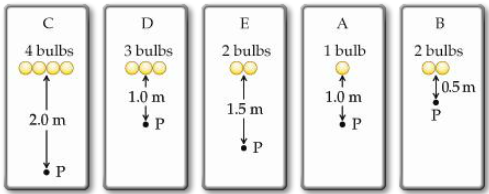# Problem: Rank the light intensity, from largest to smallest, at the point P in the figures.

###### FREE Expert Solution

Light intensity, I:

$\overline{){\mathbf{I}}{\mathbf{=}}{\mathbf{n}}\frac{\mathbf{P}}{\mathbf{4}\mathbf{\pi }{\mathbf{r}}^{\mathbf{2}}}}$

96% (356 ratings)###### Problem Details

Rank the light intensity, from largest to smallest, at the point P in the figures.Frequently Asked Questions

What scientific concept do you need to know in order to solve this problem?

Our tutors have indicated that to solve this problem you will need to apply the Energy Carried by Electromagnetic Waves concept. You can view video lessons to learn Energy Carried by Electromagnetic Waves. Or if you need more Energy Carried by Electromagnetic Waves practice, you can also practice Energy Carried by Electromagnetic Waves practice problems.

What professor is this problem relevant for?

Based on our data, we think this problem is relevant for Professor Greene's class at St. Marys University.